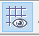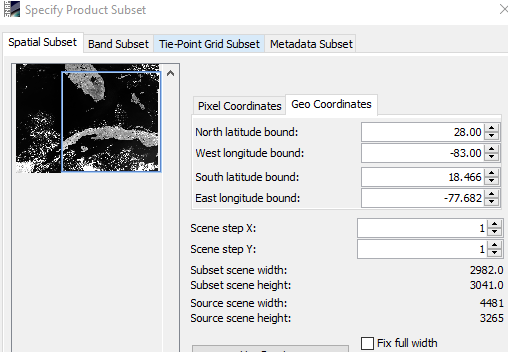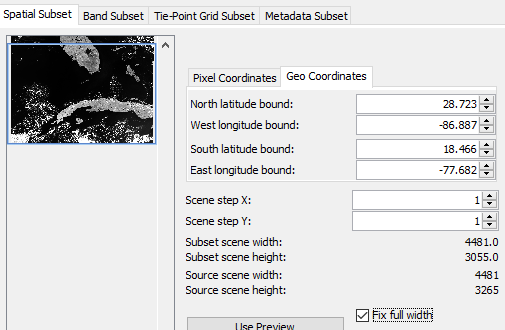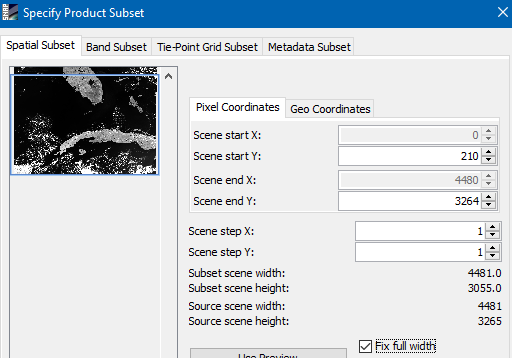# snap subset issue for MERIS

Hi,

The subset tool in SNAP 7.0 (raster -> subset) is generating unusual pixel coordinate values when I input geo-coordinates (West=-83.0, North=28.0 and fixed full width) for the following MERIS image:
MER_FRS_1PPEPA20040124_154153_000003572023_00369_09942_8978.N1
The corresponding pixel coordinates given by the subset GUI are:
Scene start Y = 993
Scene end Y = 3585

However, when I view the MERIS image in SNAP I noticed that the 28 degree North line has the following pixel coordinates: (Y=1000,X=0), (Y=575,X=2300), (Y=78,X=4480), which makes sense.
Hence the minimum pixel coordinate that the 28 degree North line is 78 on the right-hand side of the image. This would mean that,
Scene start Y = 78 (…right?)

When I run the Subset GUI (with Scene start Y = 993 and Scene end Y = 3585) I noticed that the upper right hand side of the image only goes to a latitude of 25.72 degrees North.

I’m just wondering if this is a bug.

Regards,
Rodrigo

Why do you think that the 28 degree North line has the following pixel coordinates: (Y=1000,X=0), (Y=575,X=2300), (Y=78,X=4480)?
Actually, the line leaves the scene at roughly 80°W (X=2600, Y=0). You can see it when you enable the graticule overlay.
The screenshot shows already the enhanced graticule of the next SNAP version (a little teaser). When you enable it it looks different.

If I specify 28°N and 83°W, the GUI shows this:The upper left corner of the blue rectangle is at the given 28°N, 83°W.
After marking the full-width checkbox it looks like this:.
The rectangle is just extended in width.
The latitude and longitude values are updated according to coordinates of the first pixel (scene start Y = 210 and Scene start X = 0).What might confuses you is that the text fields in the GUI for geo coordinates are named ‘bounds’. Actually, these define just the corner coordinates in the source scene.
The subset does not follow the parallels or the meridians.